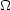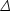1.4. The standard cosmological model

We now have most of the ingredients in place to describe the cosmological model. Beyond those of the previous subsections, there is only one parameter which is essential, which is a measure of the ionization state of the Universe. The Universe is known to be highly ionized at low redshifts (otherwise radiation from distant quasars would be heavily absorbed in the ultra-violet), and the ionized electrons can scatter microwave photons altering the pattern of observed anisotropies. The most convenient parameter to describe this is the optical depth to scattering(i.e. the probability that a given photon scatters once); in the approximation of instantaneous and complete re-ionization, this could equivalently be described by the redshift of re-ionization zion.

The basic set of cosmological parameters is therefore as shown in Table 1. The spatial curvature does not appear in the list, because it can be determined from the other parameters using Eq. (1.1). The total present matter densitym =dm +b is usually used in place of the dark matter density.

 Parameter Symbol Value Hubble parameter h 0.73 ± 0.03 Total matter densitymm h2 = 0.134 ± 0.006 Baryon densitybb h2 = 0.023 ± 0.001 Cosmological constantSee Ref.  Radiation densityrr h2 = 2.47 × 10-5 Neutrino densitySee Sec. 1.2 Density perturbation amplitude2(k*) See Ref.  Density perturbation spectral index n n = 0.97 ± 0.03 Tensor to scalar ratio r r < 0.53 (95% conf) Ionization optical depth= 0.15 ± 0.07

As described in Sec. 4, models based on these ten parameters are able to give a good fit to the complete set of high-quality data available at present, and indeed some simplification is possible. Observations are consistent with spatial flatness, and indeed the inflation models so far described automatically generate spatial flatness, so we can set k = 0; the density parameters then must sum to one, and so one can be eliminated. The neutrino energy density is often not taken as an independent parameter. Provided the neutrino sector has the standard interactions the neutrino energy density while relativistic can be related to the photon density using thermal physics arguments, and it is currently difficult to see the effect of the neutrino mass although observations of large-scale structure have already placed interesting upper limits. This reduces the standard parameter set to eight. In addition, there is no observational evidence for the existence of tensor perturbations (though the upper limits are quite weak), and so r could be set to zero. 1 More controversially, one could argue that as no evidence against the Harrison-Zel'dovich spectrum has yet been seen, then n could be set to one. We will however allow it to vary. This leaves seven parameters, which is the smallest set that can usefully be compared to the present cosmological data set. This model is referred to by various names, includingCDM, the concordance cosmology, and the standard cosmological model.

Of these parameters, onlyr is accurately measured directly. The radiation density is dominated by the energy in the cosmic microwave background, and the COBE FIRAS experiment has determined its temperature to be T = 2.725 ± 0.001 Kelvin , corresponding tor = 2.47 × 10-5 h-2.

In addition to this minimal set, there is a range of other parameters which might prove important in future as the dataset further improves, but for which there is so far no direct evidence, allowing them to be set to a specific value. We discuss various speculative options in the next section. For completeness at this point, we mention one other interesting parameter, the helium fraction, which is a non-zero parameter that can affect the microwave anisotropies at a subtle level. Presently, big-bang nucleosynthesis provides the best measurement of this parameter, and it is usually fixed in microwave anisotropy studies, but the data are just reaching a level where allowing its variation may become mandatory.

1 More controversially, one could argue that as no evidence against the Harrison-Zel'dovich spectrum has yet been seen, then n could be set to one. We will however allow it to vary. Back.